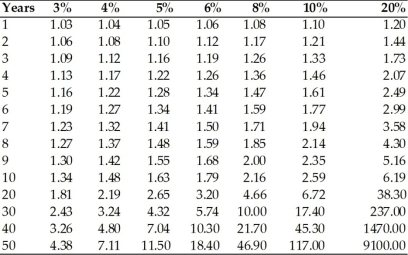• FAQ
• Contact/ Homework Answers / Economics / 51) A small reduction in a country's growth rate a
Not my Question
Flag Content

# Question

51) A small reduction in a country's growth rate is a concern to policy makers because

A) a small change can have large effects on per capita GDP over time.

B) a reduction usually leads to future reductions until finally the economy stagnates.

C) policy makers focus too much on economic growth and not enough on increasing savings rates.

D) the larger GDP is the better the economic welfare will be in the future.

52) Suppose two countries have per capita real GDP of \$20,000 in 2010. Country A has a growth rate of 4 percent and Country B has a growth rate of 5 percent. By 2013, the per capita real GDPs for the two countries, respectively, are (rounded)

A) \$21,630 and \$22,050.

B) \$22,400 and \$23,000.

C) \$22,500 and \$23,150.

D) \$25,000 and \$26,500.53) According to the above table, if per capita real GDP is currently \$1000, then at a constant annual rate of growth of 8 percent, per capita real GDP ten years from now will be equal to

A) \$2000.

B) \$2140.

C) \$2160.

D) \$2590.

54) Refer to the above table. How long would it take for a country to triple its GDP if the GDP grew at a 20 percent rate?

A) 2 years

B) 4 years

C) 6 years

D) 10 years

55) Refer to the above table. Two countries have per capita real GDPs in 2010 of \$5000. If country A has a 4 percent growth rate and Country B a 5 percent growth rate, what will the per capita real GDPs of each be in the year 2060?

A) A: \$40,000; B: \$60,000

B) A: \$35,550; B: \$57,500

C) A: \$24,000; B: \$35,200

D) A: \$15,000; B: \$30,000

56) Refer to the above table. Suppose one country has a per capita real GDP of \$1000 and another has a per capita real GDP of \$10,000, or ten times larger. If both countries have a growth rate of 5 percent, how much larger will per capita real GDP be in the second country be than the first after 50 years?

A) 4 times larger

B) 5 times larger

C) 8 times larger

D) 10 times larger

57) Refer to the above table. Country A has a per capita real GDP of \$1000 and B has a per capita real GDP of \$10,000. A is growing at a rate of 5 percent a year and B at a rate of 4 percent a year. After 50 years, how much larger is per capita real GDP in B than A? How much is this in real dollars?

A) B is 8 times larger, or \$175,000 larger on a real per capita basis.

B) B is 12 times larger, or \$230,000 larger on a real per capita basis.

C) B is a little over 6 times larger, or almost \$60,000 larger on a real per capita basis.

D) B is a little less than 2 times smaller, or almost \$20,000 smaller on a real per capita basis.

58) Refer to the above table.  If an economy's current per capita real GDP is \$3,000, and if its economy grows at an constant annual rate of 5 percent for 50 years, what will be its per capita real GDP at the end of that period?

A) \$13,140

B) \$21,330

C) \$34,500

D) \$55,200

59) Refer to the above table. You have a choice among four alternatives. Choice A lets you invest \$250,000 at 4 percent; B lets you invest \$125,000 at 6 percent; C lets you invest \$62,500 at 8 percent, and D lets you invest \$31,250 at 10 percent. Which choice will get you to \$1 million faster?

A) A

B) B

C) C

D) D

60) All of the following will cause the reported growth rate in a country to change EXCEPT

A) population changes.

B) changes in the number of poor people in the country.

C) a shift of the production possibilities curve.

D) changes in productivity.

## Solution 5 (1 Ratings )

Solved
Economics 2 Months Ago 12 Views